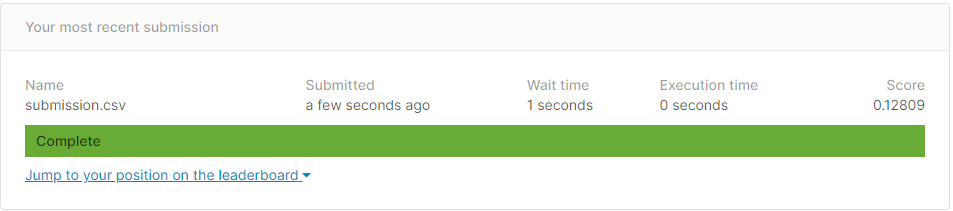# [PYTHON] Kaggle House Prices ③ ～ Forecast / Submission ～

Use the model created below to predict test data and submit a submission file. Kaggle House Prices② ~ Model Creation ~

``````import numpy as np
from sklearn.externals import joblib
``````

``````def load_x_test() -> pd.DataFrame:

:return:Test data features
"""

:return:Model of target fold
"""

:return:Predicted results of test data
"""
``````

## Predict test data

``````#Predict test data by averaging the models of each fold learned by cross-validation
preds = []
n_fold = 4

#Make predictions with each fold model
for i_fold in range(n_fold):
print(f'start prediction fold:{i_fold}')
pred = model.predict(test_x)
preds.append(pred)
print(f'end prediction fold:{i_fold}')

#Get the mean of the forecast
pred_avg = np.mean(preds, axis=0)

#Saving prediction results
joblib.dump(pred_avg, 'pred-test.pkl')
``````

## Create a submission file for submission

``````pred = load_pred_test()
print(len(pred))